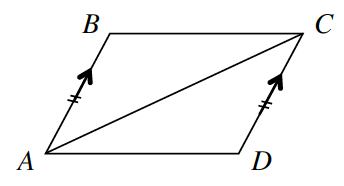### Home > INT2 > Chapter 7 > Lesson 7.1.5 > Problem7-60

7-60.

What else can you prove about quadrilaterals? Prove that if a pair of opposite sides of a quadrilateral is both congruent and parallel, then the quadrilateral must be a parallelogram. For example, for the quadrilateral $ABCD$ at right, given that $\overline{AB} // \overline{CD}$ and $\overline{AB} ≅ \overline{CD}$, show that $\overline{BC} // \overline{AD}$. Organize your reasoning in a proof (two-column or flowchart). Then record your theorem in your Theorem Graphic Organizer.If a pair of opposite sides is parallel and congruent then the quadrilateral is a parallelogram.
Use the if part of the statement as the givens, and the then part of the statement as the prove portion in the proof.

Statements

Reasons

1. $\overline{AB}//\overline{CD}$

Given

Given

1. $∠BAC ≅ ∠DCA$

Segment is congruent to itself

1. $ΔABC ≅ ΔCDA$

1. $∠BCA ≅ ∠DAC$

Alt. int. angles $≅→$ parallel

1. $ABCD$ is a parallelogram